# linear programming

(redirected from Mixed integer programming)
Also found in: Dictionary, Thesaurus, Financial, Acronyms.
Related to Mixed integer programming: Dynamic programming

## linear programming,

solution of a mathematical problem concerning maximum and minimum values of a first-degree (linear) algebraic expression, with variables subject to certain stated conditions (restraints). For example, the problem might be to find the minimum value of the expression x+y subject to the restraints x≥0, y≥0, 2x+y≥12, 5x+8y≥74, and x+6y≥24. The solution was set forth by the Russian mathematician L. V. Kantorovich in 1939 and was developed independently by the American George B. Dantzig, whose first work on the subject appeared in 1947. A faster, but more complex technique, that is suitable for problems with hundreds or thousands of variables, was developed by Bell Laboratories mathematician Naranda Karmarkar in 1983. Linear programming is particularly important in military and industrial planning.

## Linear Programming

a mathematical discipline dealing with the theory and methods of solution of extremum problems for linear functions on sets defined by systems of linear inequalities and equalities. Linear programming is a branch of mathematical programming.

A typical problem of linear programming is to maximize the linear function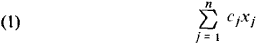subject to the constraints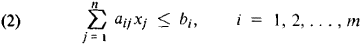(3) xj ≥ 0, j = 1, 2,..., n

where cj, aij, and bi are given variables.

Linear-programming problems are mathematical models of numerous problems that have technical and economic applications. Let us consider as an example the following problem of planning the operation of an enterprise. The production of uniform products requires the expenditure of various production factors, such as raw materials, manpower, the stock of machine tools, fuel, and transport. Usually there exist several developed technological modes of production with varying expenditures of the production factors per unit time for the production of the articles. The number of production factors used and the number of articles made depend on the amount of time the enterprise will spend on a particular technological mode of production.

The problem is then raised of efficiently distributing the working time of the enterprise among the various technological modes of production such that the maximum number of articles will be produced under the given limitations upon the cost of each production factor. We state the problem in the following manner. Suppose there exist n technological modes of producing an article and m production factors. Let us introduce the following notation. Let cj be the number of articles produced per unit time through the jth technological mode of production, aij be the expenditure of the ith production factor per unit time during the jth technological mode of production, bi be the available resources of the ith production factor, and xj be the planned working time according to the jth technological mode of production. The value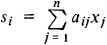denotes the total expenditure of the ith production factor under the plan x(i) = (x1(i), x2(i) ..., xn(i)). Since the resources are limited by the values bi, the conditions (2) and (3) naturally arise. The problem is posed of finding a distribution of time (optimal plan) x* = (x*1, x*2, ..., x*n) of work for each technological mode of production such that the total volume of production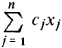is at a maximum, that is, the problem (1)–(3). The transportation problem is another example of applied linear-programming problems.

The choice of the term “linear programming” is not very apt. Linear programming is concerned with solving problems of compiling an optimal program (plan) of activities. It may therefore be considered as a mathematical method of operations research.

The function (1) in linear programming is generally called the object-function, or efficiency criterion; the vector x = (x1, x2,..., xn) is the plan; the vector x* = (x*1, x*2,..., x*n) is the optimal plan; and the set defined by the conditions (2)–(3) is the feasible set, or set of plans. The simplex method is one of the basic methods of solving linear-programming problems. Geometrically it consists in the following. The feasible set (2)-(3) is a convex polyhedral set (if bounded, a multidimensional convex polyhedron). If a linear-programming problem has a solution, there exists a vertex x* of the polyhedral set that is an optimal plan. The simplex method consists in obtaining an arrangement of the vertices in which the value of the object-function increases as we go from one vertex to the next. To each vertex there corresponds a system of equations that can be selected in a special fashion from the system of inequalities (2)-(3), and therefore the computational procedure of the simplex method consists in successively solving systems of linear algebraic equations. The simplicity of the algorithm makes this method suitable for computers.

### REFERENCE

Iudin, D. B., and E. G. Gol’shtein. Lineinoe programmirovanie. Moscow, 1969.

V. G. KARMANOV

## linear programming

[′lin·ē·ər ′prō‚gram·iŋ]
(mathematics)
The study of maximizing or minimizing a linear function ƒ(x1, … , xn ) subject to given constraints which are linear inequalities involving the variables xi .

## Linear programming

An area of mathematics concerned with the minimization (or maximization) of a linear function of several variables subject to linear equations and inequalities. The subject in its present form was created in 1947, when G.B. Dantzig defined the general model and proposed the first, and still the most widely used, method for its solution: the simplex method.

Although the linearity assumptions are restrictive, many algorithms for extensions of linear programming, such as problems with nonlinear or integer restrictions, involve successively solving linear programming problems. With a result in 1979 giving a polynomially bounded ellipsoid method, an alternative to the simplex method, linear programming became the focus of work by computer scientists, and nonlinear methods have been refocused on solving the linear programming problem. Work by N. K. Karmarkar announced in 1984 attracted much attention because of claims of vastly improved performance of a new interior method. The relative merits of Kamarkar's method and the simplex method remain to be determined, but there seems to be a place for both methods. Karmarkar's work stimulated considerable activity in linear programming methodology. See Nonlinear programming, Optimization

The linear programming problems is to minimize linear objective function (1) subject to restrictions (2).

(1)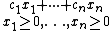(2)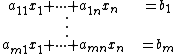The variables x1, …, xn are required to take on real values, and the coefficients aij, cj, and bi are real constants. The objective could be to maximize rather than minimize, and among constraints (2) the equations could be replaced by inequalities of the form less-than-or-equal-to or greater-than-or-equal-to. The set of xj's satisfying constraints (2) form a convex polyhedron, and the optimum value of the objective function will always be assumed at a vertex of the polyhedron unless the objective function is unbounded. The simplex method works by moving from vertex to vertex until the vertex yielding the optimum value of the objective function is reached, while interior methods stay inside the polyhedron.

An important extension in practice is to integer programming, where some of the xj's are required to take on integral values. The most common case in practice is where the integer xj must be 0 or 1, representing decision choices such as to whether to switch from production of one product to another or whether to expand a warehouse to allow for larger throughput. Whereas linear programming solution times tend to be less than an hour, adding the constraint that some or all of the xj's must be integral may cause the running time to be very long.

Early work on computer programs was done in the 1950s. Commercial computer codes implementing the simplex method have been used in industry since the mid-1960s. Efficient methods for handling the structures encountered have been developed. In particular, the matrices tend to be very sparse, that is, most (usually over 99%) of the aij's are zeroes. In the 1980s, intense development in software was begun because of changed hardware and new algorithmic developments.

Following the early work on codes, the petroleum industry quickly became the major user of linear programming, and still is an important user, especially for blending models in petroleum refining. Commercial codes are used in industry and government for a variety of applications involving planning, scheduling, distribution, manufacturing, and so forth. In universities, linear programming is taught in most business schools, industrial engineering departments, and operations research departments, as well as some mathematics departments. The model is general enough to be useful in the physical and social sciences. The improved computational efficiency achieved in the 1980s has gone hand-in-hand with expanded applications, particularly in manufacturing, transportation, and finance. See Operations research

## linear programming

(application)
A procedure for finding the maximum or minimum of a linear function where the arguments are subject to linear constraints. The simplex method is one well known algorithm.

## linear programming

A mathematical technique used to obtain an optimum solution in resource allocation problems, such as production planning.
References in periodicals archive ?
Review of studies on facility layouts Problem Method Researchers Mixed integer programming Love & Wong (1976), Amaral (2006a), Amaral (2008) Nonlinear programming Heragu & Kusiak (1991) Dynamic programming Picard & Queyranne (1981), Kouvelis & Chiang (1996) Cutting planes Amaral (2009) Semidefinite programming Anjos et al.
A summary of the important results of the mixed integer programming model is presented in Table 1.
TABLE 1 RESULTS OF THE MIXED INTEGER PROGRAMMING MODEL Five-Year Farm Plan (Years) Objective Premium Per Initial Base 1 2 3 4 5 Value Base Acre 0 CT CT PC-WS PC-WS PC-WS \$342,220 -- 33% CT CT PC-WS PC-WS PC-WS 350,131 \$23.75 50% CT PC-WS PC-WS PC-WS PC-WS 356,876 29.31 67% CT PC-WS PC-WS PC-WS PC-WS 371,614 43.87 100% PC PC PC PC PC 437,976 96.00 Notes: Results obtained for a 1,000-acre Alabama cotton farm, with market prices of \$.64/lb.
Li, "Genetic algorithm for nonlinear mixed integer programming problems and its applications," Computers and Industrial Engineering, vol.
The advanced planning and scheduling problem defined in this paper is similar to the situation in the literature  which deals with it by a mixed integer programming (MIP) method.
Ji, "A mixed integer programming model for advanced planning and scheduling (APS)," European Journal of Operational Research, vol.
Intended for students in management and industrial engineering as well as supply chain management professionals, this volume examines the use of mixed integer programming to design supply chain systems and to model complex scheduling problems.
In conventional mathematical models, the problem has been formulated as a mixed integer programming model.
(1) We develop the mixed integer programming model (MIP).
Baetz and Neebe (1994) developed a mixed integer programming model designed to optimize recycling of various by-product materials within the overall municipal solid waste management system.

Site: Follow: Share:
Open / Close